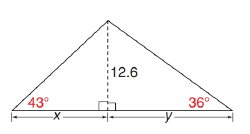Chapter 11.3, Problem 44E### Elementary Geometry for College St...

6th Edition
Daniel C. Alexander + 1 other
ISBN: 9781285195698

#### Solutions

Chapter
Section### Elementary Geometry for College St...

6th Edition
Daniel C. Alexander + 1 other
ISBN: 9781285195698
Textbook Problem
1 views

# In the triangle shown, find each measure to the nearest tenth of a unit. a) x b) y c) A , the area of the triangleTo determine

a)

The value of side x to the nearest tenth of a unit.

Explanation

Given:

The given figure is shown below:

Calculation:

The triangles ABC are right triangles.

According to the definition of tangent ratio, tan(mBCA)=ABBC

tan(mBCA)=ABBC=12

To determine

b)

The value of side y to the nearest tenth of a unit.

To determine

c)

The area of the triangle of the given figure.

### Still sussing out bartleby?

Check out a sample textbook solution.

See a sample solution

#### The Solution to Your Study Problems

Bartleby provides explanations to thousands of textbook problems written by our experts, many with advanced degrees!

Get Started

#### In Exercises 13-20, sketch a set of coordinate axes and plot each point. 20. (1.2, 3.4)

Applied Calculus for the Managerial, Life, and Social Sciences: A Brief Approach

#### limx(lnx)1/x= a) 0 b) 1 c) e d)

Study Guide for Stewart's Single Variable Calculus: Early Transcendentals, 8th

#### True or False: converges.

Study Guide for Stewart's Multivariable Calculus, 8th

#### Define fraud and explain the safeguards that exist to prevent it.

Research Methods for the Behavioral Sciences (MindTap Course List)